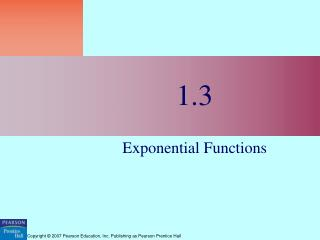DownloadDownload Presentation1.3

# 1.3

Download Presentation## 1.3

- - - - - - - - - - - - - - - - - - - - - - - - - - - E N D - - - - - - - - - - - - - - - - - - - - - - - - - - -
##### Presentation Transcript

1. 1.3 Exponential Functions

2. What you’ll learn about… • Exponential Growth • Exponential Decay • Applications • The Number e …and why Exponential functions model many growth patterns.

3. Rules for Exponents

4. Exponential Function

5. Exponential Growth

6. Exponential Growth If 0 < a < 1, then the graph of f looks like the graph of y = 2-x = .5x.

7. Compound Interest The formula for calculating the value of an investment that is awarded annual compound interest is T=P(1+r)twhere T is the total value of the investment, P is the principal, r is the rate of interest (as a decimal) and t is the number of years.

8. Calculate the value of a 3 year certificate of deposit given the initial investment was \$500 and the CD earns 2% annually.

9. If an investment earns 4% annually, how long will it take for the value of the investment to double?

10. Half-life Exponential functions can also model phenomena that produce decrease over time, such as happens with radioactive decay. The half-life of a radioactive substance is the amount of time it takes for half of the substance to change from its original radioactive state to a non-radioactive state by emitting energy in the form of radiation.

11. Exponential Growth and Exponential Decay The function y = k ● ax, k > 0, is a model for exponential growth if a > 1, and a model for exponential decay if 0 < a < 1.

12. Example Exponential Functions Use a graphing calculator to find the zero’s of f(x)= 4x – 3.

13. The Number e

14. The Number e The exponential functions y = exand y = e-x are frequently used to model exponential growth or decay. Interest compounded continuously uses the model y = P ert, where P is the initial investment, r is the interest rate (as a decimal) and t is the time in years.

15. Example The Number e • The approximate number of fruit fies in an experimental population after t hours is given by Q(t) = 20e0.03t, t ≥ 0. • Determine the initial number of flies in the poplation. • How large is the population after 72 hours? • Graph the function. [0,100] by [0,120] in 10’s

16. Quick Review

17. Quick Review

18. Quick Quiz Sections 1.1 – 1.3

19. Quick Quiz Sections 1.1 – 1.3

20. Quick Quiz Sections 1.1 – 1.3 3. The half-life of a certain radio active element is 8 hours. There are 5 grams present initially. Which of the following gives the best approximation when there will be 1 gram remaining? • 2 • 10 • 15 • 16 • 19

21. Assignment pages 26 – 28, 3-21 multiples of 3, 23-27, 32, 36, 40